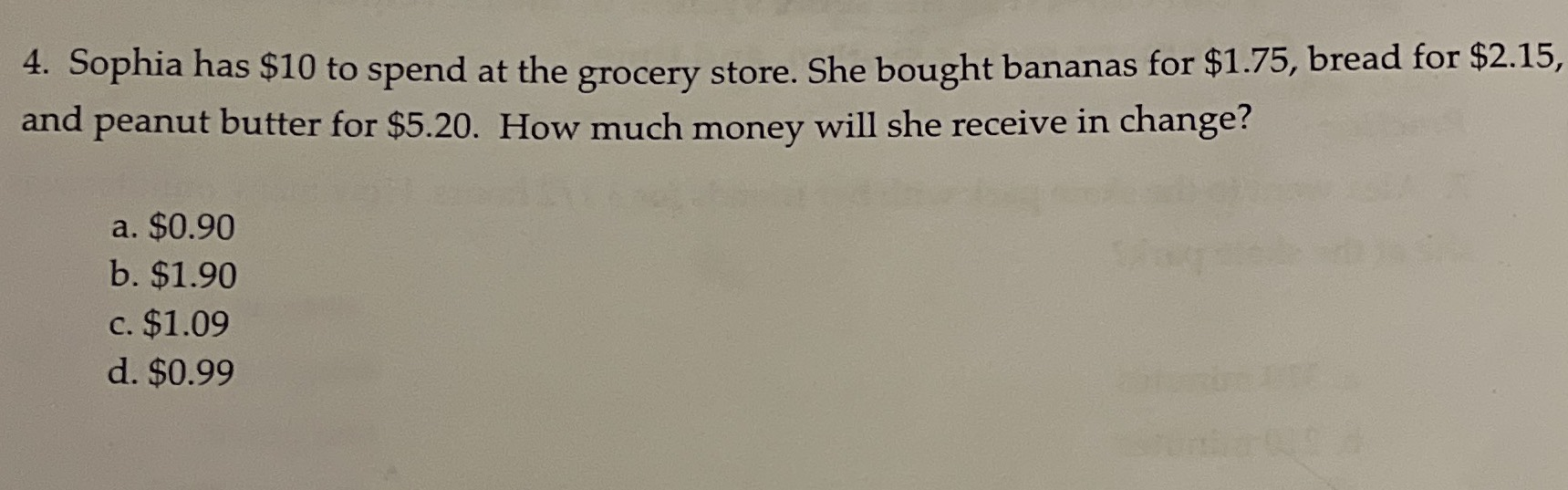### ¿Todavía tienes preguntas de matemáticas?

Pregunte a nuestros tutores expertos
Algebra
Pregunta4. Sophia has $$\ 10$$ to spend at the grocery store. She bought bananas for $$\ 1.75$$ , bread for $$\ 2.15$$ , and peanut butter for $$\ 5.20$$ . How much money will she receive in change? a. $$\ 0.90$$

b. $$\ 1.90$$

c. $$\ 1.09$$

d. $$\ 0.99$$